## Charge Conjugate Waves

Assume that, in addition to rotation, boost and parity symmetry, the Dirac equation also has a symmetry under charge conjugation. We wish to write the Dirac equation in a way that makes the symmetry between electron and positron clear. Start from the Dirac equation and include the coupling to the EM field with the substitution that.The strategy is to try to write the charge conjugate of this equation then show that it is equivalent to the Dirac equation with the right choice of charge conjugation operator for. First of all, the sign of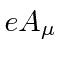is expected to change in the charge conjugate equation. (Assume the equation, including the constantis the same but the sign of the EM field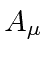changes.) Second assume, for now, that the Dirac spinor is transformed to its charge conjugate by the operation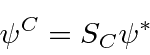where we are motivated by complex scalar field experience.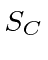is a 4 by 4 matrix. The charge conjugate equation then isTake the complex conjugate carefully remembering thatandwill change signs.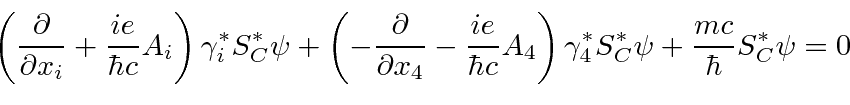Multiply from the left by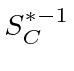.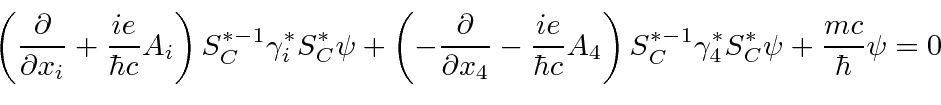Compare this to the original Dirac equation,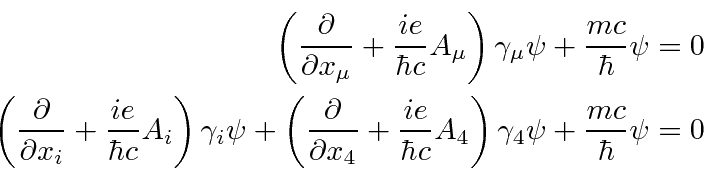The two equations will be the same if the matrixsatisfies the conditions.Recalling thematrices in our representation,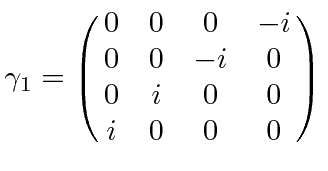note that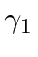andare completely imaginary and will change sign upon complex conjugation, whileandare completely real and will not. The solution in our representation (only) isIt anti-commutes withandproducing a minus sign to cancel the one from complex conjugation. It commutes withgiving the right + sign. It anti-commutes withgiving the right - sign.

The charge conjugate of the Dirac spinor is given by.Of course a second charge conjugation operation takes the state back to the original.

Applying this to the plane wave solutions gives.The charge conjugate of an electron state is the negative energy'' electron state, the absence of which would produce a positron of the same energy, momentum, spin, and velocity as the electron.That is, the conjugate is the hole needed to make a positron with the same properties as the electron except that it has opposite charge.

Let us take one more look at a plane wave solution to the Dirac equation, for exampleand its charge conjugate, from the point of view that a positron is an electron moving backward in time. Discard the idea of the negative energy'' sea. Assume that we have found a new solution to the field equations that moves backward in time rather than forward.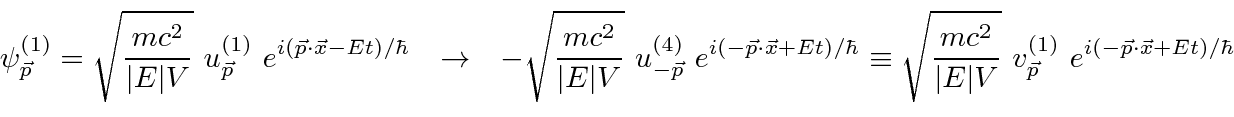The charge conjugate of the electron solution is an electron with the same charge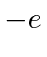, opposite momentum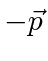, and spin opposite to the original state. It satisfies the equation with the signs of the EM fields reversed and, because the sign of theterm in the exponential is reversed, it behaves as a positive energy solution moving backward in time, with the right momentum and spin.

Our opinion of the negative energy'' solutions has been biased by living in a world of matter. We know about matter waves oscillating as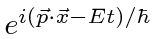. There is a symmetric set of solutions for the same particles moving backward in time'' oscillating as. These solutions behave like antiparticles moving forward in time. Consider the following diagram (which contributes to Thomson scattering) from two points of view. From one point of view, an electron starts out at, lets say in the state. At time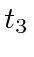, the electron interacts with the field and makes a transition to the statewhich travels backward in time towhere it again interacts and makes a transition to. From the other point of view, the electron starts out at, then, at time, the field causes the creation of an electron positron pair both of which propagate forward in time. At time, the positron and initial electron annihilate interacting with the field. The electron produced atpropagates on into the future.No reference to the negative energy'' sea is needed. No change in the negative energy'' solutions is needed although it will be helpful to relabel them with the properties of the positron rather than the properties of the electron moving backward in time.

The charge conjugation operation is similar to parity. A parity operation changes the system to a symmetric one that also satisfies the equations of motion but is different from the original system. Both parity and charge conjugation are good symmetries of the Dirac equation and of the electromagnetic interaction.The charge conjugate solution is that of an electron going backward in time that can also be treated as a positron going forward in time.

Jim Branson 2013-04-22